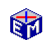The Classical Model

The Classical Model builds on the principles developed in microeconomics to explain how equilibrium production and employment might be determined from profit maximizing and utility maximizing behavior.Model Link:  The Classical Model
Printable PDF Exercises

The EconModel presentation of the Classical Model first goes over the various model elements:

• Production
• Labor Demand
• Labor Supply
• Aggregate Supply and Demand
• Loanable Funds

You then trace out movements in the equilibrium for these markets given the following changes:

• Technology Shocks (view screenshot)
• Taxes on Labor Income
• Monetary and Fiscal Policy

Movie:  The Classical Model (40 seconds)

In addition to illustrating the properties of the Classical Model, these exercises provide the background against which the Keynesian models were developed.

Classic Economic Models

Macroeconomics

Introduction
Overview of Macro Models

Microeconomics

Introduction
Overview of Micro Models

Resources